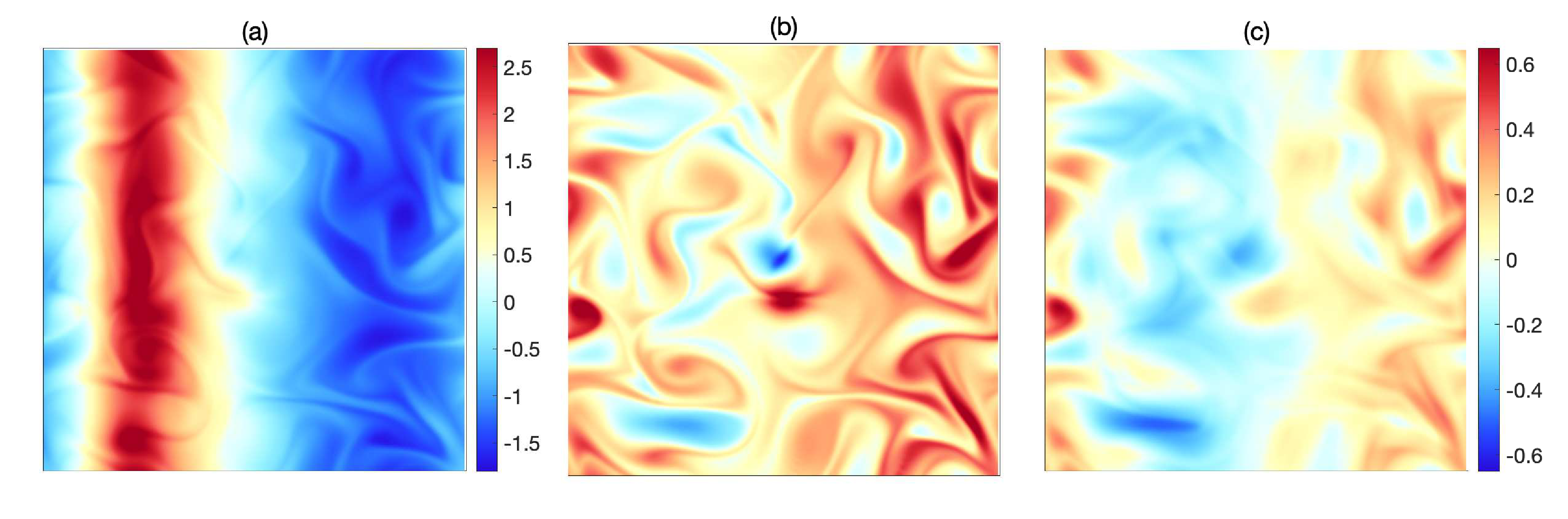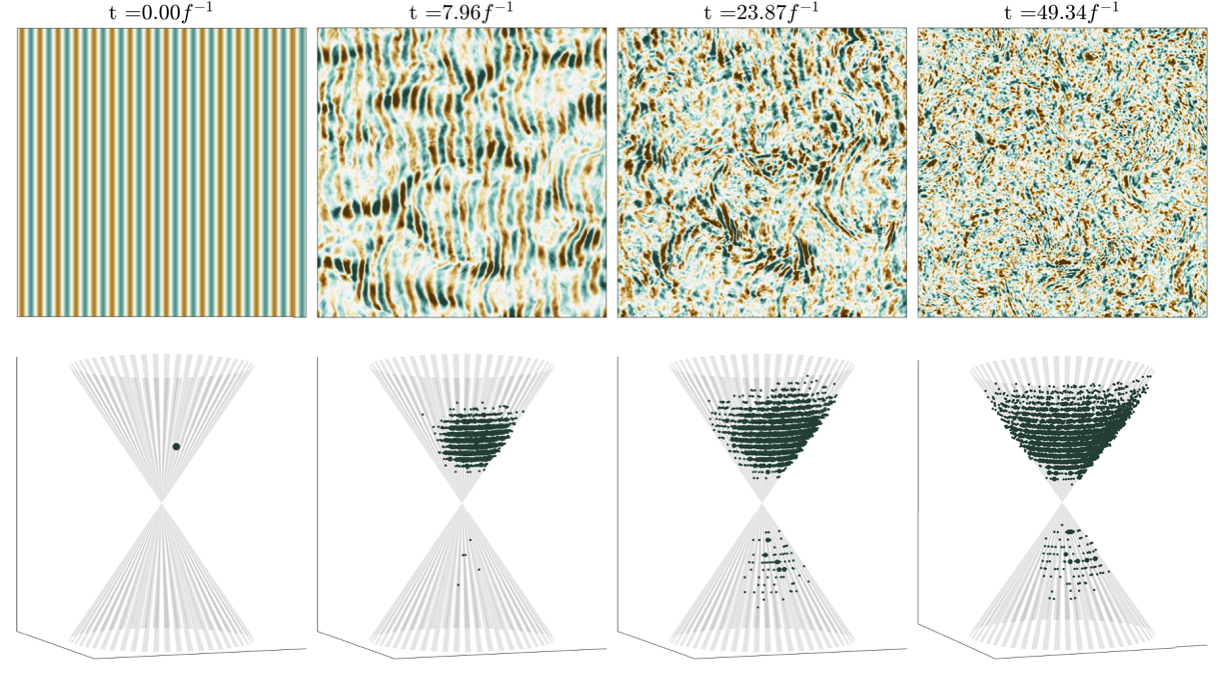## Computing Lagrangian Means Without Particle TrackingVorticity field and its Lagrangian and Eulerian means in a shallow-water simulation: (a) instantaneous vorticity, (b) Lagrangian mean vorticity, and (c) Eulerian mean vorticity. Panels (b) and (c) share the same colourbar shown to the right of panel (c). More details in Kafiabad & Vanneste 2022.
Time averaging is an essential tool in analysing multi-timescale flows and can be performed in two ways: 1) Eulerian and 2) Lagrangian. The Eulerian mean is the average of flow variables at fixed spatial points, whereas the Lagrangian mean is the average of flow variables along particle trajectories. Lagrangian averaging has several pivotal advantages over its Eulerian counterpart. One of these advantages is demonstrated in the figure above, where a high-amplitude wave is interacting with turbulent shallow water. The strong wave – present in the instantaneous vorticity field (panel (a)) – is filtered out after both types of averaging. However, Lagrangian averaging (panel (b)) has preserved the small-scale features of the flow that are blurred by Eulerian averaging (panel (c)). Lagrangian averaging also removes the Doppler shift that eclipses the separation of time scales between the background flow and waves leading to a more reliable decomposition of the two. Despite its advantages, the widespread adoption of Lagrangian averaging has been hindered by computational complications. To compute the Lagrangian mean in numerical models usually particles are tracked using interpolated velocities at particle positions at every time steps, which requires substantial memory usage and is ill suited for efficient computational parallelisation.
We propose a novel numerical method to compute Lagrangian means that does not require tracking particles and consquently circumvents these difficulties. In this approach, we compute the Lagrangian mean as solutions to a set of Partial Differential Equations (PDEs) integrated over the averaging interval. This paradigm could be a breakthrough in computing Lagrangian means as these PDEs can be discretised in a variety of ways and solved on-the-fly (i.e. simultaneously with the dynamical governing equations). Hence, they do not require storing any time series and substantially reduce the memory footprint compared to particle tracking.

Early version of this work is presented in this talk for the University of Hamburg.

## Scattering of Inertia-Gravity Waves by Geostrophic Turbulence

Two horizontal slices of 3D Boussinesq simulation initiated by geostrophic turbulence superimposed with a monochromatic plane wave. Left: total vertical vorticity, right: wave's vertical velocity.

Inertia-gravity waves generated from different sources such as tides, topography and convection scatter as they travel through the large-scale atmospheric and oceanic vortices. This scattering process is shown in the above video where a monochromatic plane wave is superimposed with geostrophic turbulence in a 3D Boussinesq simulation. As it can be seen, the wave field (shown in the right panel) eventually loses its preferred direction and develops small scale features. The interaction of these waves with the slowly moving vortices has very important implications in the energetics and the dynamics of geophysical flows. Relying on the statistical isotropy and stationarity of vortices and their temporal (and spatial) scale separation with waves, we have put forward a theory that describes the evolution of wave energy in Fourier space. More specifically, it predicts that the waves energy diffuses on constant frequency surfaces, which are double cones in 3D Boussinesq flows (see the figure below). When the forced waves reach a steady state, this theory shows that the wave energy spectrum scales as $k-2$ (k being the wavenumber). This provides a possible answer to the long-standing question of the mechanism underpinning the observed atmospheric spectra.

Considering it a breakthrough, the Journal of Fluid Mechanics selected our work for the Focus on Fluid article, where an invited expert wrote the following review about our findings:

Young. “Inertia-gravity waves and geostrophic turbulence.” Journal of Fluid Mechanics, 920, 2021.Scattering of a plane inertia-gravity wave by a turbulent geostrophic flow. Top row is the vertical velocity field for z = 0, and bottom row is the representation of the wave energy distribution in Fourier space at four successive times (increasing from the left to right). The constant-frequency cone associated with the frequency of initial plane wave is shown by the stripes; small-, medium- and large-sized dots indicate wave energy density exceeding, respectively, 0.004%, 0.02% and 0.2% of the initial wave energy.

## Efficient Computation of Wave Transport and Scattering

Atmospheric and oceanic (internal and surface) waves typically have wavelengths that are smaller than the grid size of operational numerical models; as a result, they cannot be fully resolved. Fortunately, the phase of these waves is irrelevant to their impact, and it is sufficient to model their phase-averaged properties that are felt at the grid size of numerical models. This process of phase-averaging, however, leads to a set of new equations that depend on three-dimensional wavenumbers in addition to three-dimensional spatial coordinates. The high-dimensionality of these equations poses a new computational challenge. In this project, we address this challenge by applying the mathematical technique termed dynamical low-rank approximation to reduce the e ective dimensionality of the problem. This approach has a great competitive edge over the alternatives, because it judiciously reduces the computations and can be parallelised very efficiently . This is a work in progress.

## Interaction of Near-Inertial Waves with Vortices

Horizontal slices of a 3D Boussinesq simulation initialised by a strong inertial wave and an anticyclone. Left: the wave kinetic energy. Right: total vertical vorticity.

Near-inertial waves (NIWs) are generated at the ocean surface and are one of the main reservoirs of kinetic energy in the ocean. Hence, their interaction with the eddies and vortices are very important. Anticyclonic vortices focus and trap these waves so that near-inertial energy levels are elevated within the vortex core. This is demonstrated in the video above, which is a simulation of a high-amplitude inertial wave interacting with an anticyclone. The left panel shows the wave energy in a horizontal slice of this flow and the right shows the vertical vorticity at the same slice which is affected by waves oscillations. The waves kinetic energy is periodically trapped in this vortex in a time scale much slower than the inertial period (i.e. the wave period). We investigate some aspects of this process, including the nonlinear modification of the vortex by the wave and the frequency of wave trapping, using analytical tools which are compared against the high-resolution simulation of the Boussinesq equations.

## Breakdown of Balance Dynamics from a Turbulence Perspective

Vertical vorticity at a horizontal and a vertical slice of 3D Boussinesq simulation.Only a part of domain is shown.

Balance models (such as geostrophic balance) reduce the full equations to a simpler set to describe large-scale atmospheric and oceanic flow. Their solutions, however, can spontaneously generate imbalance in the context of the full equations. To study this phenomenon, we perform high-resolution turbulence simulations of the non-hydrostatic Boussinesq equations, starting with a high-order nonlinearly balanced initial condition (which is the next-order improvement to quasigeostrophic). The above videos is an example of Boussinesq simulation (only a part of domain shown), which shows that imbalance is generated in time in some regions of this flow. By increasing the dimensionless parameters such as Rossby and Froude, we then investigate at what scales balance breaks down and after how long. Our results show that the balanced initial condition displays a steep geostrophic energy spectrum and an even steeper ageostrophic energy spectrum. However, a shallow tail later develops in the ageostrophic energy spectrum at small scales if Ro and the Reynolds number, Re, are large enough. This shallow range moves toward smaller wavenumbers if Ro is increased.

A part of this project is presented in this talk for the Banff International Research Station.